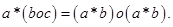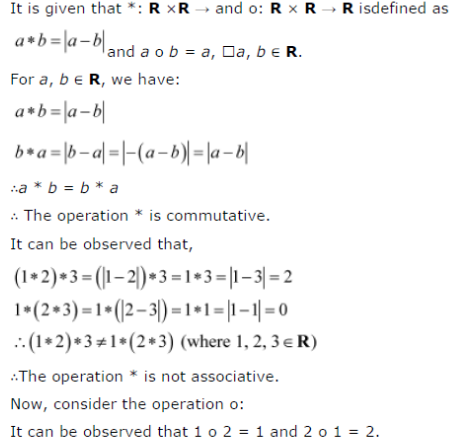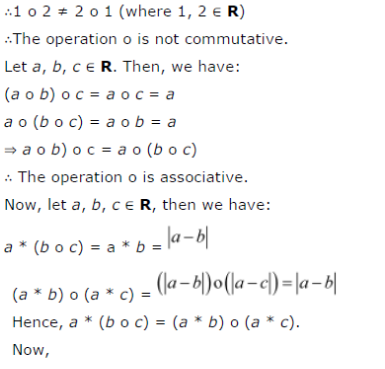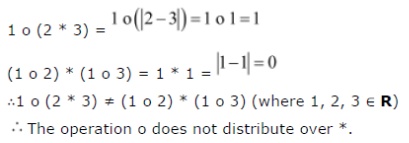# Consider the binary operation * : R x R a * (boc) = (a * b) o(a * b)

Consider the binary operation * : R x RR and o = R x RR defined asandR. Show that * is commutative but not associative, o is associative but not commutative. Further, show thatR,[If it is so, we say that the operation * distributes over the operation o]. Does o distribute over *? Justify your answer.